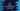# C# program to check if an item exists in an array## C# program to check if an item exists in an array:

In this post, we will learn how to check if an item exists in an array or not in C#. We can use a loopfor that, but I will show you a different way to check that.

Array.Exists method can be used to check if an item is in an array or not. This method takes one array and one predicate. It uses the predicate and based on its finding it returns one boolean value or true/false.

### Definition of Array.Exists:

This method is defined as below:

``public static bool Exists<T> (T[] array, Predicate<T> predicate);``

This is a static method.

Here, T is the type of element of the array.

array is the given one-dimensional array, where we are checking. predicate is the Predicate that is used to check in the array elements.

It returns one Boolean value. true if one or more elements are matched by the predicate. Else false.

It throws ArgumentNullException if the array or predicate is null.

### Example of Array.Exists:

Let’s take a look at the below example:

``````using System;

public class Program

{

​	public static void Main()

​	{

​		int[] firstArr = new int[]{11,21,31,41,50};
​		int[] secondArr = new int[]{11,31,41,71,91};
​		int[] thirdArr = new int[]{20,41,60,81,10};

​		Console.WriteLine(Array.Exists(firstArr, e => e%5 == 0));
​		Console.WriteLine(Array.Exists(secondArr, e => e%5 == 0));
​		Console.WriteLine(Array.Exists(thirdArr, e => e%5 == 0));

​	}

}``````

Here,

• firstArr, secondArr, and thirdArr are three integer arrays given.
• We are using Array.Exists to check if these arrays contain any value that is divisible by 5.
• The first Console checks for the firstArr, the second Console checks for the secondArr, and the third Console checks for the thirdArr.

If you run the above program, it will print the below output:

``````True
False
True``````

### Predicate as a separate function:

We can also write the Predicate as a separate function. This helps us to do complex checking in the function and we can simply pass the function name as the second argument.

For example, we can write the above program as like below:

``````using System;

public class Program

{

​	static bool isDivisibleByFive(int value){

​		return value % 5 == 0;

​	}

​	public static void Main()

​	{

​		int[] firstArr = new int[]{11,21,31,41,50};
​		int[] secondArr = new int[]{11,31,41,71,91};
​		int[] thirdArr = new int[]{20,41,60,81,10};

​		Console.WriteLine(Array.Exists(firstArr, isDivisibleByFive));
​		Console.WriteLine(Array.Exists(secondArr, isDivisibleByFive));
​		Console.WriteLine(Array.Exists(thirdArr, isDivisibleByFive));

​	}

}``````

It will give the same output.